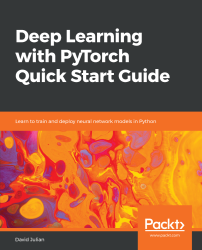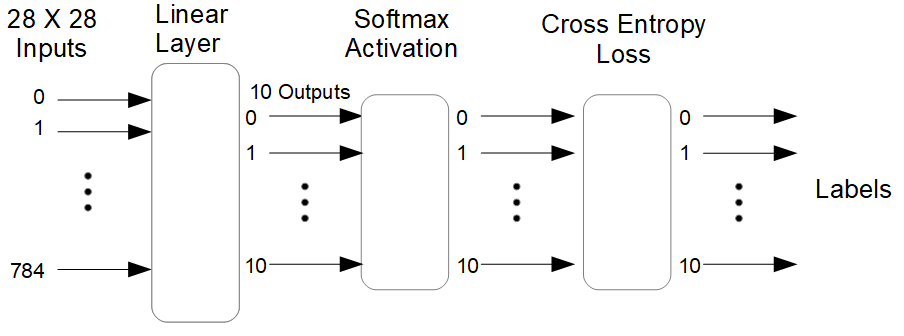•#### Deep Learning with PyTorch Quick Start Guide#### Overview of this book

PyTorch is extremely powerful and yet easy to learn. It provides advanced features, such as supporting multiprocessor, distributed, and parallel computation. This book is an excellent entry point for those wanting to explore deep learning with PyTorch to harness its power. This book will introduce you to the PyTorch deep learning library and teach you how to train deep learning models without any hassle. We will set up the deep learning environment using PyTorch, and then train and deploy different types of deep learning models, such as CNN, RNN, and autoencoders. You will learn how to optimize models by tuning hyperparameters and how to use PyTorch in multiprocessor and distributed environments. We will discuss long short-term memory network (LSTMs) and build a language model to predict text. By the end of this book, you will be familiar with PyTorch's capabilities and be able to utilize the library to train your neural networks with relative ease.
PrefaceFree Chapter
Introduction to PyTorchDeep Learning FundamentalsComputational Graphs and Linear ModelsConvolutional NetworksOther NN ArchitecturesGetting the Most out of PyTorchOther Books You May Enjoy# Multi-class classification example

So far, we have been using trivial examples to demonstrate core concepts in PyTorch. We are now ready to explore a more real-world example. The dataset we will be using is the MNIST dataset of hand-written digits from 0 to 9. The task is to correctly identify each sample image with the correct digit.

The classification model we will be building consists of several layers and these are outlined in the following diagram:The images we are working with are 28 x 28 pixels in size, and each pixel in each image is characterized by a single number, indicating its gray scale. This is why we need 28 x 28 or 784 inputs to the model. The first layer is a linear layer with 10 outputs, one output for each label. These outputs are fed into to the softmax activation layer and cross-entropy loss layer. The 10 output dimensions represent the 10 possible classes...